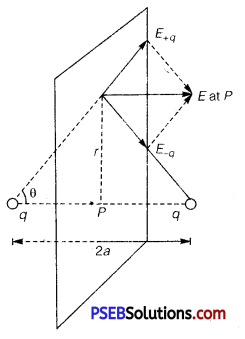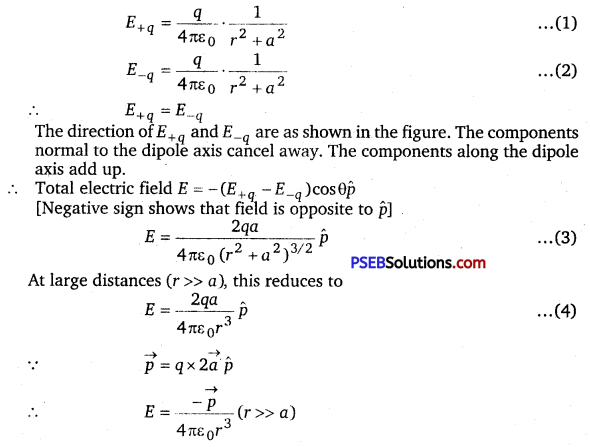# PSEB 12th Class Physics Important Questions Chapter 1 Electric Charges and Fields

Punjab State Board PSEB 12th Class Physics Important Questions Chapter 1 Electric Charges and Fields Important Questions and Answers.

## PSEB 12th Class Physics Important Questions Chapter 1 Electric Charges and Fields

Question 1.
Define electrostatics.
Electrostatics deals with the study of forces, fields and potentials arising from static charges.

Question 2.
Define charge.
It is defined as the basic and characteristic property of elementary particles of matter in the form of which certain force of interaction energies may be explained.Question 3.
What are the behaviour of charges?
Like charges repel and unlike charges attract each other.

Question 4.
Define the polarity of charge.
The property which differentiates the two kinds of charges is called the polarity of charge.

Question 5.
Who first assigned the positive and negative signs to charge?
Benjamin Franklin

Question 6.
What are conductors?
The substances which allow electricity to pass through them easily are called conductors e.g., metals, human, earth etc.

Question 7.
What are insulators?
Most of the non-metals like glass, porcelain, plastic, nylon, wood offer high resistance to the passage of electricity through them, they are called insulators.Question 8.
Define the semiconductors.
The substances which offer resistance to the movement of charges are called semiconductors. They are intermediate between conductors and insulators,

Question 9.
What are point charges?
If the sizes of charged bodies are very small as compared to the distances between them, they are called point charges.

Question 10.
Write the law of conservation of charges.
“The total charge of the isolated system is always conserved.” It is not possible to create or destroy net charge carried by isolated system although the charge carrying particles may be created or destroyed in a process.

Question 11.
The dimensions of an atom are of the order of an Angstrom. Thus there must be large electric fields between the protons and electrons. Why then is the electrostatic field inside a conductor zero? (NCERT Exemplar)
The electric fields bind the atoms to neutral entity. Fields are caused by excess charges. There can be no excess charge on the inner surface of an isolated conductor. So, the electrostatic field inside a conductor is zero.

Question 12.
An arbitrary surface encloses a dipole. What is the electric flux through this surface? (NCERT Exemplar)
Net charge on a dipole = -q + q = 0. According to Gauss’s theorem, electric flux through the surface,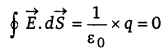Question 13.
Sketch the electric field lines for a uniformly charged hollow cylinder shown in figure. (NCERT Exemplar)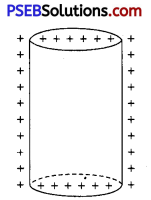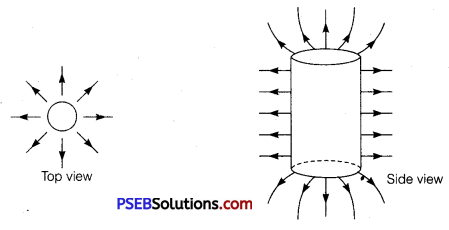Question 1.
Describe the gold-leaf electroscope.
Question
A simple apparatus to detect charge on a body is the gold-leaf electroscope. It consists of a vertical metal rod housed in a box, with two thin gold leaves attached to its bottom end. When a charged object touches the metal knob at the top of the rod, charge flows on to the leaves and they diverge. The degree of divergence is an indicator of the amount of charge.

Question 2.
Define the grounding or earthing.
When we bring a charged body in contact with the earth, all the excess charge on the body disappears by causing a momentary current to pass to the ground through the connecting conductor (such as our body). This process of sharing the charges with the earth is called grounding or earthing.

Question 3.
What is the importance of earthing in buildings?
Earthing provides a safety measure for electrical circuits and appliances. A thick metal plate is buried deep into the earth and thick wires are drawn from this plate; these are used in buildings for the purpose of earthing near the mains supply. The electric wiring in our houses has three wires; live, neutral and earth. The first two carry electric current from the power station and the third is earthed by connecting it to the buried metal plate. Metallic bodies of the electric appliances such as electric iron, refrigerator, TV are connected to the earth wire. When any fault occurs or live wire touches the metallic body the charge flows to the earth without damaging the appliance and without causing any injury to the humans; this would have otherwise been unavoidable since the human body is a conductor of electricity.Question 4.
Describe the additivity of charges in brief.
If a system contains two point charges q1 and q2, the total charge of the system is obtained simply by adding algebraically qi and q2, i. e., charges add up like real numbers or they are scalars like the mass of a body. If a system contains n charges q1, q2, q3,……, qn, then the total charge of the system is q1 + q2 + q3 + … + qn. Charge has magnitude but no direction, similar to the mass. However, there is one difference between mass and charge. Mass of a body is always positive whereas a charge can be either positive or negative. Proper signs have to be used while adding the charges in a system. For example, the total charge of a system containing five charges +1, +2, -3, +4 and -5, in some arbitrary unit, is (+1) + (+2) + (-3) + (+4) + (-5) = -1 in the same unit.

Question 5.
(a) Define electric flux. Write its SI unit.
(b) A small metal sphere carrying charge +Q is located at the centre of a spherical cavity inside a large uncharged metallic spherical shell as shown in the figure. Use Gauss’s law to find the expressions for the electric field at points P1 and P2.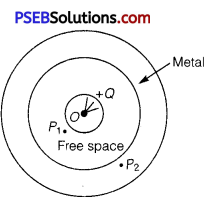(c) Draw the pattern of electric field lines in this arrangement.
(a) Electric flux over an area in an electric field represents the total number of electric field lines crossing this area and is given by the product of surface area and the component of electric field intensity normal to the area.
The SI unit of flux is Nm2C-1.

(b) Let point P1 is at distance R from the centre 0.
S1 is the Gaussian surface, then according to Gauss’s theorem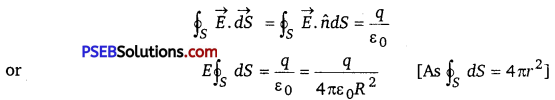Inside the shell the charge is zero, so the field is also zero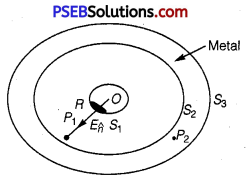(c) The direction of electric field is shown in figure.

Question 6.
A metallic spherical shell has an inner radius R1 and outer radius R2– A charge Q is placed at the centre of the spherical cavity. What will be surface charge density on (i) the inner surface, and (ii) the outer surface? (NCERT Exemplar)
When a charge +Q is placed at the centre of spherical cavity,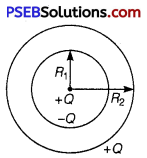The charge induced on the inner surface = -Q
The charge induced on the outer surface = +Q
∴ Surface charge density on the inner surface = $$\frac{-Q}{4 \pi R_{1}^{2}}$$
Surface charge density on the outer surface = $$\frac{+Q}{4 \pi R_{2}^{2}}$$Question 7.
Two charges q and -3q are placed fixed on x-axis separated by distance id\ Where should a third charge 2q be placed such that it will not experience any force? (NCERT Exemplar)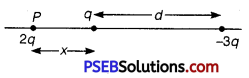Let the charge 2q be placed at point P as shown. The force due to q is to the left and that due to -3q is to the right.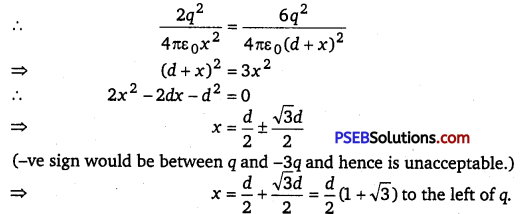Question 1.
How can you charge a metal sphere positively without touching it?
Figure (a) shows an uncharged metallic sphere on an insulating metal stand. Bring a negatively charged rod close to the metallic sphere, as shown in Fig. (b). As the rod is brought close to the sphere, the free electrons in the sphere move away due to repulsion and start piling up at the farther end. The near end becomes positively charged due to deficit of electrons. This process of charge distribution stops when the net force on the free electrons inside the metal is zero. Connect the sphere to the ground by a conducting wire. The electrons will flow to the ground while the positiye charges at the near end will remain held there due to the attractive force of the negative charges on the rod, as shown in Fig. (c). Disconnect the sphere from the ground. The positive charge continues to be held at the near end [Fig.(d)]. Remove the electrified rod. The positive charge will spread uniformly over the sphere as shown in Fig. (e).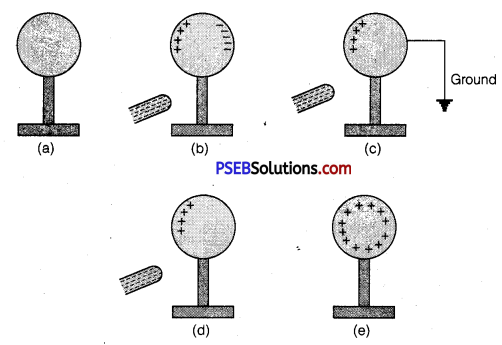In this experiment, the metal sphere gets charged by the process of induction and the rod does not lose any of its charge.

Similar steps are involved in charging a metal sphere negatively by induction, by bringing a positively charged rod near it. In this case the electrons will flow from the ground to the sphere when the sphere is connected to the ground with a wire.Question 2.
Define electric dipole moment. Is it a scalar or a vector? Derive the expression for the electric field of a dipole at a point on the equatorial plane of the dipole.
In vector form it is written as , where the direction of  is from negative charge to positive charge.